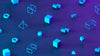# What is Symmetry?

This maths fact blog is all about symmetry. If you want to know more about 2D shapes or 2D shape angles make sure you read our collections of maths blogs.

## What is a line of symmetry?

A line of symmetry is a line that cuts a shape exactly in half. The shape is exactly the same on both sides of the line.

All of these shapes have lines of symmetry drawn on them:

## What is reflective symmetry?

Reflective symmetry is when a shape is exactly the same on both sides of the line. If we put a mirror on the line of symmetry we would be able to see the whole shape.

Download and print out this reflective symmetry worksheet. Draw the lines of symmetry on each shape. Hint: Some shapes may not have any lines of symmetry.

## What is rotational symmetry?

Rotational symmetry and reflective symmetry are different.

A shape has rotational symmetry if it can be rotated around a centre point and fit into itself. Each time during a rotation that a shape fits into itself it is called an order.

Download and print this rotational symmetry shape worksheet. Work out how many rotational symmetry orders each shape has. Hint: Some shapes may not have any orders of rotational symmetry.

## When is symmetry taught in the Maths National Curriculum?

Symmetry is taught as part of the geometry strands of the maths curriculum. These strands are geometry - properties of shapes

Symmetry is first taught in year 2 when children:

Primary school children revisit symmetry in year 4 when they:

### Related Posts